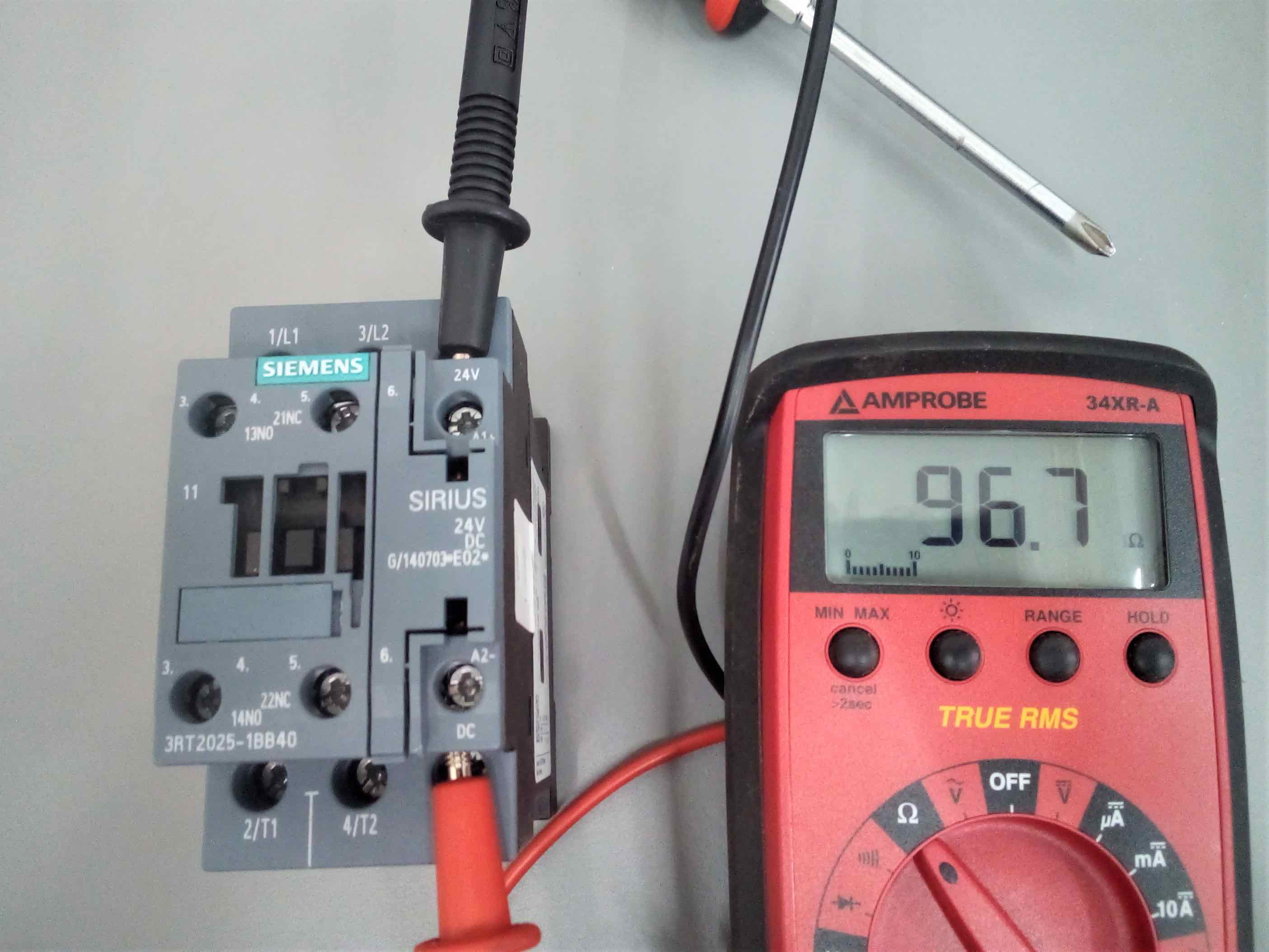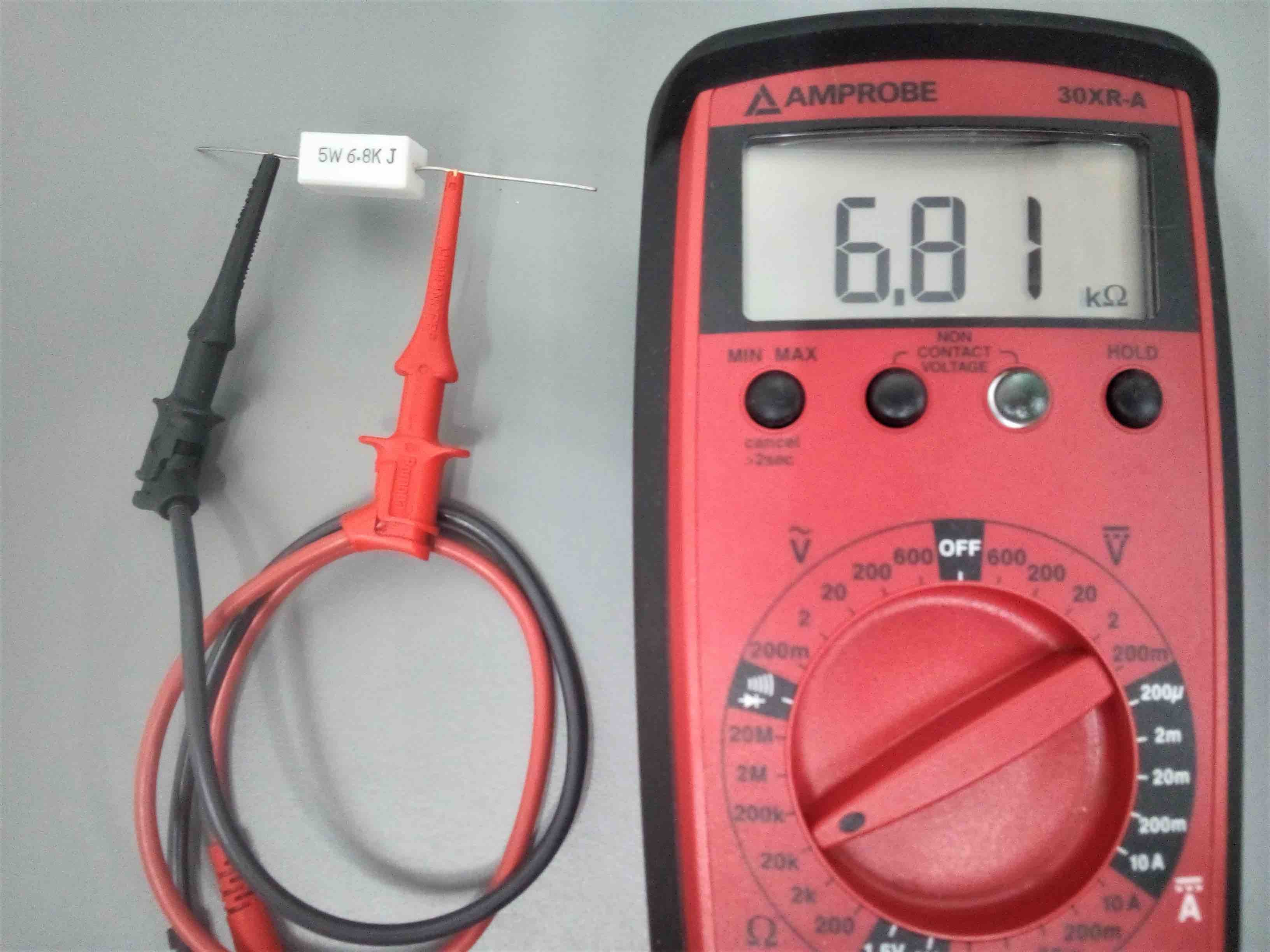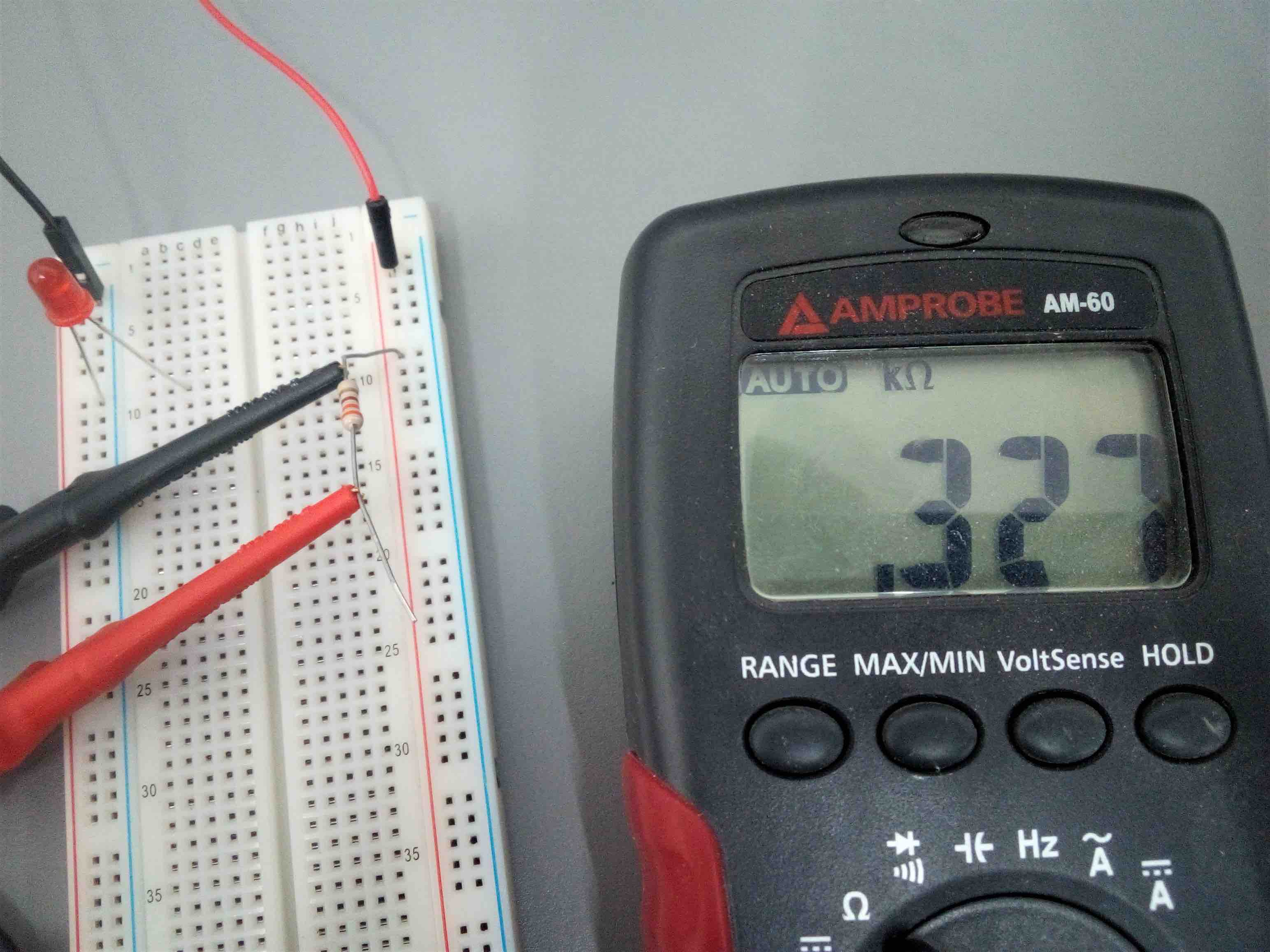Technical Article

# How to Measure Resistance with a Multimeter

June 09, 2020 by David Peterson

## When the power is removed to a circuit, the common measurements of voltage and current prove meaningless. The only remaining option is to use resistance testing to determine which components and conductors are working.### What is Resistance?

In most situations, a measurement of voltage or current is most useful to track down failure in a control circuit. Voltage requires only that power is applied, the load does not need to be active. Current does require the circuit to have electricity actively flowing, but clamp-type meters allow this to be a simple measurement.

However, if the power must be removed from the circuit then neither of these two measurements can properly show any information and an alternative must be used.

Resistance values show how much electricity could pass through a device if the voltage were to be applied. A resistance, measured in Ohms, is the relationship between voltage and current. High resistance leads to low current, low resistance leads to high current.##### A resistance test of a contactor shows a coil resistance of around 97 Ohms. According to the part datasheet, the value should be 98 Ohms, this one works well.

A resistance value of zero, or nearly zero is considered a ‘short’ circuit and can cause a fuse or breaker to trip with excessive current. At the other extreme, a very high resistance value which the meter shows as OL (or ‘over limit’) is an ‘open’ circuit and the current will be nearly nothing.

### Benefits of Resistance

When used properly, the resistance value can actually tell more than voltage or current alone can show. In a control circuit, voltage always tends to be lost either at the load device or at the first point at which the circuit is ‘open’. This may be an open switch or a broken wire, among other reasons.

But from any of these sources, the voltage drop will be the full source voltage - it does not give us any indication of how much electricity is flowing. In fact, an open switch with full voltage will lead to zero current flow, while a load device with full voltage drop will be some non-zero current flow, even though the measurement is the same.

Resistance allows a technician to verify two separate facts about the circuit. First, if the voltage were to be applied, the current will flow. If some amount of resistance exists in the circuit, then we can say with certainty that some current will be flowing when the circuit is properly connected and powered.

The second fact learned from resistance is the value of how much current will be flowing when power is applied. This is a fact that voltage measurements cannot determine, so in some cases, resistance may be more important than voltage alone. If a relay coil has partially failed, it may still drop all of the source voltage just like before, but the current draw may be different. Resistance could prove that the relay will conduct some current, and it may show exactly how much current will flow.

According to Ohm’s law, for a control circuit with a single load, simply divide the source voltage (24 v, or 120v, etc) by the measured resistance. This will be the resulting current.##### A resistance test placed on a power resistor verifies that the printed value is correct. This resistor is also rated to withstand 5 watts of power dissipation.

Since power must be removed to use make resistance tests, it’s important to understand the function of the meter in order to make proper tests that show the correct information.

### Cautions Against External Voltage

The first important thing to understand is that the meter develops its own voltage to send electricity through the test device. This voltage is from the battery. The test device drops some voltage, and the meter internally drops the remainder with a series voltage divider. Using the ratio of internal fixed-resistance and measured voltage versus the battery voltage, the test device resistance can easily be calculated and displayed.

However, if the leads are placed on a live circuit, the internal measured resistance may be more than the battery source voltage. This ‘impossible’ scenario is shown on the display as a negative value. If you see a negative value shown on the screen, remove the leads immediately. It likely has not damaged the meter, but a high voltage can be damaging. If the meter is manually-ranging, the lowest resistance scales will be the most likely to be damaged by an external voltage.

To give absolute peace of mind that you can prevent this external voltage problem, simply remove one of the wires leading to the device. This will ensure that the device cannot be a part of the active circuit.### Solid State Devices

The other caution that deserves emphasis with all modern electronics is the attempt to test solid-state devices. In purely resistive circuits, the phrase ‘linear’ means a constant resistance for all voltages - raise the voltage and the current will also increase at the same rate. However, for solid-state devices like diodes and transistors, the amount of applied voltage may change the resistance.

Common sense can be very misleading here because the device under test may show a legitimate resistance value when removed from the circuit, leading the technician to believe the device is working properly. However, when returned to the circuit and the circuit voltage is different, this may drastically alter the resistance. In these cases, it’s important to use voltage tests when the circuit is live for solid-state testing. Often, a small value resistor is placed in series with a solid-state device. Measure the voltage of the resistor, and along with its voltage, current can also be calculated.

Like using multimeters? We do too.

So much so that we published a downloadable eBook: The Guide to Digital Multimeters

Here are the other articles in this multimeter series: